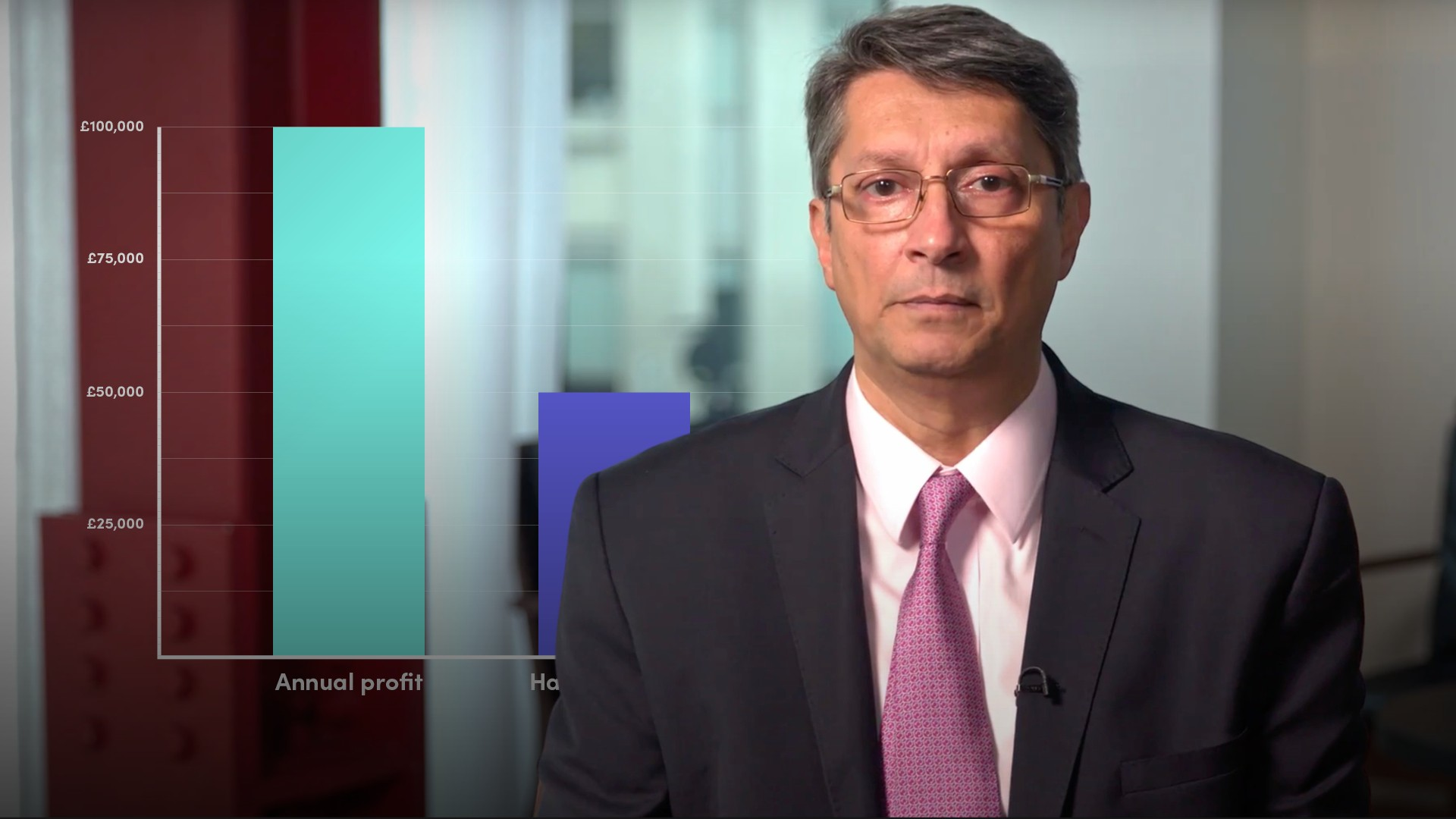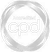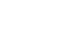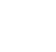# Calculating Discrete Holding Period Returns### Abdulla Javeri

As investors or traders, the main objective is to make a profit. Returns can be calculated for a holding period or on an annualised basis, and on a continuously compounded basis or a discrete basis. In this video, Abdulla will provide a formula for calculating returns on a discrete basis for both the holding period and an annual percentage return.

Financial MathsAs investors or traders, the main objective is to make a profit. Returns can be calculated for a holding period or on an annualised basis, and on a continuously compounded basis or a discrete basis. In this video, Abdulla will provide a formula for calculating returns on a discrete basis for both the holding period and an annual percentage return.

Speak to an expert

Speak to an expert today to access this and all of the content on our platform.### Calculating Discrete Holding Period Returns

4 mins 37 secs

Overview

Calculating returns can be expressed as a percentage gain or loss on an investment. Investors main objective is to make a profit - a positive return on their investment. These returns can be calculated using the formulae outlined below.

Key learning objectives:

• Describe the basic properties of calculating returns

• Identify the relevant formulae for calculating holding period returns and annualised returns

## Speak to an expert

Speak to an expert today to access this and all of the content on our platform.

Summary

#### What are some of the basic properties of calculating returns?

• Expressed as a percentage gain or loss on an investment
• Can be calculated for the holding period, or, on an annualised basis
• Can be expressed as a discrete return, or, on a continuously compounded basis

#### How do we calculate holding period returns?

Holding Period Return = Sell price / Buy price - 1

Or

Holding Period Return = (Sell price - Buy price) / Buy price

For a price series

Holding Period Return = End value / Start value - 1

1. The holding period return is simply the selling price divided by the buying price minus 1.
2. Or, looking at the middle formula, it’s the profit as a percentage of the cost.
3. If you currently hold an investment and you want to calculate the periodic returns since you made the investment, simply take a price (end value), divided by the previous price (start value) and subtract 1.

#### Using the information below, how do we calculate the holding period for both investors?

• Investor A - has made \$100,000 in one year
• Investor B - has made \$50,000 in six months
1. The holding period for Investor A is 10%, 100,000/1,000,000
2. The holding period for Investor B is 50%, 50,000/100,000

Investor B has a greater return in a shorter time, and thus has clearly done better.

#### How do we calculate annualised returns?

If the holding period is less than a year, we use the top formulae. And if the holding period is greater than a year, we use the bottom one.

Annual Return = Holding period return x 365 / holding period (days)

Or

Annual Return = (Sell price / Buy price)(1/years held) - 1

(Multi-period TVM formula re-arranged to solve for r)

#### For the following examples, what is the holding period return and annual return?

Question 1:
• Sell Price = 265
• Holding Period = 30 days
1. Holding period return: (265/250) - 1 = 6%
2. Annual return: (6 x 365)/30 = 73%
Question 2:
• You bought shares 10 years ago for 150, and you sold them for 650.
1. Holding period return: (650/150) - 1 = 333.3%
2. Annualised return - As the holding period is greater than one year, we do the following calculation: (650/150)(1/10) - 1 = 15.79%

## Speak to an expert

Speak to an expert today to access this and all of the content on our platform.### Abdulla Javeri

Abdulla’s career in the financial markets started in 1990 when he entered the trading floor of the London International Financial Futures Exchange, LIFFE, and qualified as a pit trader in equity and equity index options. In 1996, Abdulla became a trainer for regulatory qualifications and then for non-exam courses, primarily covering all major financial products.

There are no available videos from "Abdulla Javeri"

### Accredited by